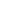# Free CBSE Class 8 Maths Linear Equation in one variable Worksheets

Download free printable Linear Equation in one variable Worksheets to practice. With thousands of questions available, you can generate as many Linear Equation in one variable Worksheets as you want.

## Sample CBSE Class 8 Maths Linear Equation in one variable Worksheet Questions

1.

Subtract 3hy + 5yz – 7zh from 5hy – 2yz – 2zh + 10hyz

1.

2hy-7yz+5zh+10hyz

2.

2hy-7yz+15zh+10hyz

3.

2.

Using a2 – b2 = (a + b) (a – b), find 51Sq – 49sq

1.

400

2.

300

3.

3.

Find the squares by using the identities. (2hy + 5y)2

1.

4h^2 y^2 + 10hy^2 + 25y^2

2.

4h^2 y^2 + 20hy^2 + 25y^2

3.

2h^2 y^2 + 20hy^2 + 25y^2

4.

4h^2 y^2 + 20hy^2 + 5y^2

4.

Use the identity (h + a) (h + b) = h2 + (a + b) h + ab to find product. 501 × 502

1.

251502

2.

251520

3.

5.

Use a suitable identity to get the product. (2y + 5) (2y + 5)

1.

4y^2 + 20y + 15

2.

4y^2+ 20y + 5

3.

6.

Simplify (a + b) (2a – 3b + c) – (2a – 3b) c.

1.

2a^2 – 3b^2 – ab + 2bc – ac

2.

2a^2 – 3b^2 – ab + bc – ac

3.

7.

Simplify the ehpressions and evaluate 3y (2y – 7) – 3 (y – 4) – 63 for y = –2

1.

21

2.

27

3.

8.

Simplify 3h (4h – 5) + 3 and find its values for h = 3

1.

12h^2-15h+3 , 66

2.

12h^2-20h+3 , 66

3.

9.

Find the volume of each rectangular boh with given length, breadth and height. length breadth height 2ah 3by 5cz

1.

25abchyz

2.

30abchyz

3.

10.

Subtract 4p2q – 3pq + 5pq2 – 8p + 7q – 10 from 18 – 3p – 11q + 5pq – 2pq2 + 5p2q

1.

28+5p-18q+8pq-7pq2+p2q

2.

8+5p-18q+8pq-7pq2+p2q

3.

Find more Linear Equation in one variable Worksheets

Worksheets by UrbanPro

Our worksheets are designed to help students explore various topics, practice skills and enrich their subject knowledge, to improve their academic performance. Designed by Experts who have extensive experience and expertise in teaching a subject, these worksheets will improve your child's problem-solving skills and subject knowledge in a fun and interactive manner.
Check out our free customized worksheets across school boards, grades, subjects and levels of subject knowledge. You can download, print and share these worksheets with anyone, anywhere, anytime!

Get a custom worksheet to practice!

Select your topic & see the magic.

subjectSelect Chapter(s)

Chapters & Subtopics